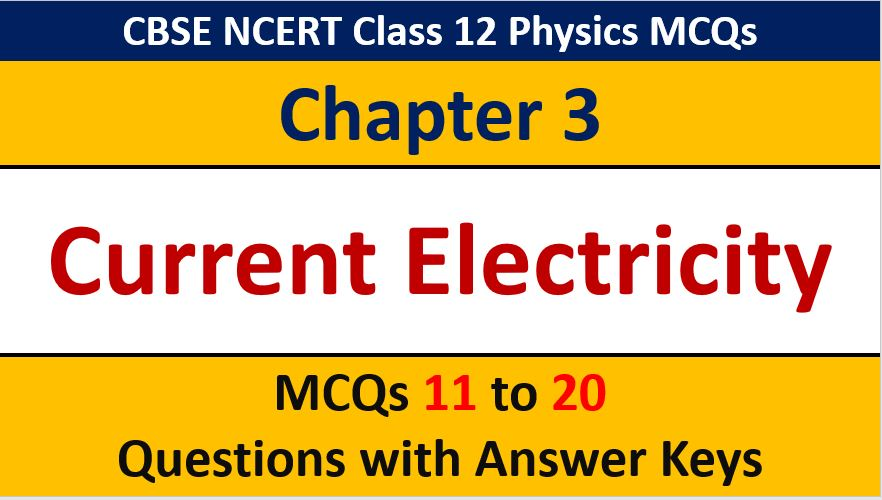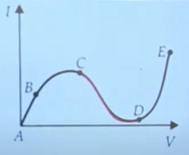## Current Electricity CBSE Class 12 Physics MCQ Question with Answer Keys Solutions

If you are preparing for CBSE Class 12 Board Exam Term 1 which will be an objective type questions paper, then you need to practice multiple choice questions of Class 12 Physics Chapterwise. In this article of AKVTutorials, you will get Current Electricity CBSE Class 12 Physics MCQ Question with Answer Keys Solutions

MCQ Questions for Class 12 Physics Chapter 3 Question No 11:

If a wire of resistivity 𝜌 is stretched to double its length, then its new resistivity will

Option A : be half

Option B : be double

Option C : be 4 times

Option D : not change

Option D : not change

MCQ Questions for Class 12 Physics Chapter 3 Question No 12:

A wire of resistance R is stretched to twice of its original length. Its new resistance will be

Option A : 4 R

Option B : R / 9

Option C : 3 R

Option D : R / 3

Option A : 4 R

MCQ Questions for Class 12 Physics Chapter 3 Question No 13:

The colour sequence in a carbon resistor is red, brown, orange, and silver. The resistance of the resistor is

Option A : 21 × 103 𝛺 ± 10 %

Option B :  23 × 103 𝛺 ± 10%

Option C :  21 × 103 𝛺 ± 5 %

Option D :  12 × 103 𝛺 ± 5 %

Option A : 21 × 103 𝛺 ± 10 %

MCQ Questions for Class 12 Physics Chapter 3 Question No 14:

The resistance of a conductor is having a following coding : green, orange, black, gold. The resistance of the resistance of the resistor is

Option A : 53 × 100 ± 5 %

Option B : 53 × 101 ± 5 %

Option C : 53 × 100 ± 10 %

Option D : 53 × 10  ± 10 %

Option C : 53 × 100 ± 5 %

MCQ Questions for Class 12 Physics Chapter 3 Question No 15:

From the graph between current / and voltage V, identify the portion corresponding to the negative resistanceOption A : AB

Option B : BC

Option C : CD

Option D : DE

Option C : CD

MCQ Questions for Class 12 Physics Chapter 3 Question No 16:

Electric field (E) and current density (j) hav relation

Option A : E 𝛂 j-1

Option B : E 𝛂 j

Option C : E 𝛂 1/j2

Option D : E2 𝛂 1/j

Option B : E 𝛂 j

MCQ Questions for Class 12 Physics Chapter 3 Question No 17:

The mobility of charge carries increases with

Option A : increase in the average collision time

Option B : increase in the electric field

Option C : increase in the mass of the charge carriers

Option D : decrease in the charge of the mobile carriers

Option A : increase in the average collision time

MCQ Questions for Class 12 Physics Chapter 3 Question No 18:

The resistivity of an alloy is ρ alloy and that of the constituent metals are ρ metal then

Option A : ρalloy < ρmetal

Option B : ρalloy = ρmetal

Option C : ρalloy > ρmetal

Option D : There is no such relation between ρalloy and ρmetal

Option C : ρalloy > ρmetal

MCQ Questions for Class 12 Physics Chapter 3 Question No 19:

Drift velocity vvaries with the intensity of the electric field as per the relation

Option A : vd E

Option B : vd 1 / E

Option C : vd = constant

Option D : vd E2

Option A : vd α E

MCQ Questions for Class 12 Physics Chapter 3 Question No 20:

Two resistors of resistances Rand R(R< R) are connected in parallel. For equivalent resistance R, the correct statement is

Option A : R > R1 +R2

Option B : R2 < R < R

Option C : R2 < R < R1 + R2

Option D : R < R2

Option D : R < R2

CBSE Class 12 Physics Chapterwise MCQ
1 : Electric Charge & Fields MCQs
| 1 to 10 | 11 to 20
2 : Electrostatics Potential and Capacitance MCQs
| 1 to 10 | 11 to 20
3 : Current Electricity MCQs
| 1 to 10 | 11 to 20
4 : Moving charges and Magnetism MCQs
| 1 to 10 | 11 to 20
5 : Magnetism and Matter MCQs
| 1 to 10 | 11 to 20
6 : Electromagnetic Induction MCQs
| 1 to 10 | 11 to 20
7 : Alternating Current MCQs
| 1 to 10 | 11 to 20
8 : Electromagnetic Waves MCQs
| 1 to 10 | 11 to 20
9 : Ray Optics and Optical Instruments MCQs
| 1 to 10 | 11 to 20
10 : Wave Optics MCQs
| 1 to 10 | 11 to 20
11 : Dual Nature of Radiation MCQs
| 1 to 10 | 11 to 20
12 : Atoms MCQs
| 1 to 10 | 11 to 20
13 : Nuclei MCQs
| 1 to 10 | 11 to 20
14 : Semi Conductor Electronics MCQs
| 1 to 10 | 11 to 20
15 : Communication Systems MCQs
| 1 to 10 | 11 to 20